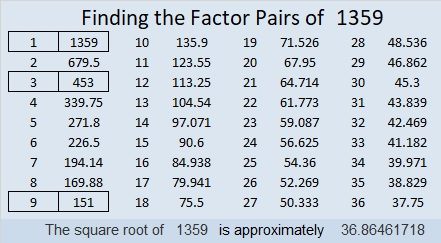# 1359 and Level 3

The first row with a clue has a 6 in it. Use that 6 and another clue that goes with it, to figure out where to put the factors of 6 in this puzzle. Then work your way down the puzzle, row by row until you have found all the factors of this level 3 puzzle.Print the puzzles or type the solution in this excel file: 12 Factors 1357-1365

Here are a few facts about the number 1359:

• 1359 is a composite number.
• Prime factorization: 1359 = 3 × 3 × 151, which can be written 1359 = 3² × 151
• 1359 has at least one exponent greater than 1 in its prime factorization so √1359 can be simplified. Taking the factor pair from the factor pair table below with the largest square number factor, we get √1359 = (√9)(√151) = 3√151
• The exponents in the prime factorization are 2 and 1. Adding one to each exponent and multiplying we get (2 + 1)(1 + 1) = 3 × 2 = 6. Therefore 1359 has exactly 6 factors.
• The factors of 1359 are outlined with their factor pair partners in the graphic below.1359 is the difference of two squares in three different ways:
680² – 679² = 1359
228² – 225² = 1359
80² – 71² = 1359

This site uses Akismet to reduce spam. Learn how your comment data is processed.